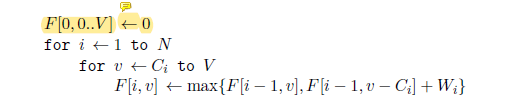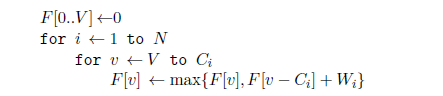• 01背包问题的具体描述自行百度： 有N件物品和一个容量为V的背包。第i件物品的重量是c[i]，价值是w[i]。 求解将哪些物品装入背包可使这些物品的重量总和不超过背包容量，且价值总和最大。 (01背包中这些物品...
01背包问题的具体描述自行百度：

有N件物品和一个容量为V的背包。第i件物品的重量是c[i]，价值是w[i]。 求解将哪些物品装入背包可使这些物品的重量总和不超过背包容量，且价值总和最大。
(01背包中这些物品每种都只有1个，每个物品只能装一次)
基本问题
用子问题定义状态：即f[i][v]表示前i件物品恰放入一个容量为v的背包可以获得的最大价值。 则其状态转移方程便是：f[i][v]=max{ f[i-1][v], f[i-1][v-c[i]]+w[i] }。可以压缩空间，f[v]=max{f[v],f[v-c[i]]+w[i]}
这个方程非常重要，基本上所有跟背包相关的问题的方程都是由它衍生出来的。所以有必要将它详细解释一下：

“将前i件物品放入容量为v的背包中”这个子问题，若只考虑第i件物品的策略（放或不放），那么就可以转化为一个只牵扯前i-1件物品的问题。

如果不放第i件物品，那么问题就转化为“前i-1件物品放入容量为v的背包中”，价值为f[i-1][v]；如果放第i件物品，

那么问题就转化为“前i-1件物品放入剩下的容量为v-c[i]的背包中”，

此时能获得的最大价值就是f [i-1][v-c[i]]再加上通过放入第i件物品获得的价值w[i] 即f[i-1][v-c[i]]+w[i]。

为什么逆序
逆序的关键就在于这个状态转移方程
f[i][v]只与f[i-1][v]和f[i-1][v-C[i]]有关，即只和i-1时刻状态有关，所以我们只需要用一维数组f[]来保存i-1时的状态f[]。 假设i-1时刻的f[]为{a0，a1，a2，…，av}，那么i时刻的f[]中第v个应该为max(av,av-C[i]+W[i])即max(f[v],f[v-C[i]]+W[i])，
这就需要我们遍历V时逆序遍历，这样才能保证求i时刻f[v]时f[v-C[i]]是i-1时刻的值。如果正序遍历则当求f[v]时 其前面的f,f，…，f[v-1]都已经改变过，里面存的都不是i-1时刻的值，这样求f[v]时利用f[v-C[i]]必定是错的值。最后f[V]即为最大价值。
数组遍历有时候必须逆序大多是这个原因。
展开全文• (可以参考下百度百科 只是我觉得百度百科对于为什么逆序这个问题解释的不是特别清楚) （以下题目中的内容摘自百度百科） /////////////////////////////////////////////////////////////////////////////////////...
首先 什么是01背包问题？(可以参考下百度百科 只是我觉得百度百科对于为什么逆序这个问题解释的不是特别清楚)
（以下题目中的内容摘自百度百科） / 题目 有N件物品和一个容量为V的背包。第i件物品的重量是c[i]，价值是w[i]。 求解将哪些物品装入背包可使这些物品的重量总和不超过背包容量，且价值总和最大。
(01背包中这些物品每种都只有1个，每个物品只能装一次)
基本思路 这是最基础的背包问题，特点是：每种物品仅有一件，可以选择放或不放。
用子问题定义状态：即f[i][v]表示前i件物品恰放入一个容量为v的背包可以获得的最大价值。 则其状态转移方程便是：f[i][v]=max{ f[i-1][v], f[i-1][v-c[i]]+w[i] }。可以压缩空间，f[v]=max{f[v],f[v-c[i]]+w[i]}
这个方程非常重要，基本上所有跟背包相关的问题的方程都是由它衍生出来的。所以有必要将它详细解释一下： “将前i件物品放入容量为v的背包中”这个子问题，若只考虑第i件物品的策略（放或不放），那么就可以转化为一个只牵扯前i-1件物品的问题。 如果不放第i件物品，那么问题就转化为“前i-1件物品放入容量为v的背包中”，价值为f[i-1][v]；如果放第i件物品， 那么问题就转化为“前i-1件物品放入剩下的容量为v-c[i]的背包中”， 此时能获得的最大价值就是f [i-1][v-c[i]]再加上通过放入第i件物品获得的价值w[i] 即f[i-1][v-c[i]]+w[i]。
注意f[v]有意义当且仅当存在一个前i件物品的子集，其费用总和为v。所以按照这个方程递推完毕后， 最终的答案并不一定是f[N] [V]，而是f[N][0..V]的最大值。
今天想了一下午01背包问题。。。  以下是我自己总结出来的  20140224
先举个例子做个小实验(该实验可以证明顺序推v是错的)：                      i          i-1             i-1 原式子(二维的):  f[i][v]=max{f[i-1][v],f[i-1][v-c[i]]+w[i]} 现在要改成一维的(空间优化):     f[v]=max{ f[v],f[v-c[i]]+w[i] }
注意上面的状态转移方程两边的是2个状态(左边的是这一状态  右边的是上一状态（二维的通过i可以看出来）)
f[i][v]是由f[i-1][v-c[i]]推出来的,现在要把二维的改成一维的,即要推f[v],要保证f[v]由f[v-c[i]]推出来， 如果v是顺序递增的,则相当于f[i][v]变得是由f[i][v-c[i]]推出来的,而不是由原来的f[i-1][v-c[i]]推的（到这里 也许你知道了原因 但可能和我当初一样没真正弄懂 那么请继续看完）(下一个状态应由上一个状态来获得)
------------------------------------------------------------------ 这里可以举个非常简单的例子（我就不像某些网友举列那么多数字的例子了 我搞个容易看懂的吧）的方法来证明v顺序递增是不行的
设有3件物品 ,背包能容纳的总重量为10 i=1,2,3
物品号         重量(c)          价值(w) i=1             4                 5
i=2             7                 9
i=3             5                 6
f[v]=max{ f[v],f[v-c[i]]+w[i] }
如果v是顺序递增 i=1时，v=4~10 （因为v要至少大于等于c[i]嘛 不然减出个负数没意义）                                                                     原先的:  f=0 f=0 f=0 f=0 f=0 f=0 f=0 f=0 f=0 f=0  f=0 ---------------------------  i=1  --------------------------------  后来的： f=0 f=0 f=0 f=0 f=5 f=5 f=5 f=5 f=0 f=0  f=0 v=4: f=max{f,f+5}    max{0,5}=5   f=5
v=5: f=max{f,f+5}    max{0,5}=5   f=5
v=6: f=max{f,f+5}    max{0,5}=5   f=5
v=7: f=max{f,f+5}    max{0,5}=5   f=5
v=8: f=max{f,f+5}    max{0,10}=10  f=10  (这里显然不对,这时i=1，只能放一件物品,然而没有一个物品的价值为10的 )
v=9: f=max{f,f+5}    max(0,10}=10  f=10
v=10: f=max{f,f+5}  max{0,10}=10  f=10   ---------------------------  i=1  -------------------------------- 既然顺序 i=1都不对 i=2和3就不用看了 由此看来顺序不行
=================================================================================
下面再来看看逆序的 我为了方便看 把上面的数据复制过来 设有3件物品 ,背包能容纳的总重量为10 i=1,2,3
物品号         重量(c)          价值(w) i=1             4                 5
i=2             7                 9
i=3             5                 6
f[v]=max{ f[v],f[v-c[i]]+w[i] }
如果v是逆序,v=10~4                 ---------------------------  i=1  --------------------------------             f=0 f=0 f=0 f=0 f=5 f=5 f=5  f=5   f=5 f=5  f=5 v=10: max{f,f+5}     max{0,5}=5      f=5
v=9: max{f,f+5}      max{0,5}=5      f=5
v=8: max{f,f+5}      max{0,5}=5      f=5
v=7: max{f,f+5}      max={0,5}=5     f=5
v=6: max{f,f+5}      max={0,5}=5     f=5
v=5: max{f,f+5}      max={0,5}=5     f=5
v=4: max{f,f+5}      max={0,5}=5     f=5
---------------------------  i=1  --------------------------------  ///
---------------------------  i=2  --------------------------------       f=0 f=0 f=0 f=0 f=5 f=5 f=5  f=9   f=9 f=9  f=9 v=10: max{f,f+9}      max{5,9}=9   f=9
v=9: max{f,f+9}      max{5,9}=9    f=9
v=8: max{f,f+9}      max{5,9}=9    f=9
v=7: max{f,f+9}      max{5,9}=9    f=9

---------------------------  i=2  --------------------------------  ///
---------------------------  i=3  --------------------------------     f=0 f=0 f=0 f=0 f=5 f=5 f=5  f=9   f=9 f=9  f=9 v=10: max{f,f+6}     max{9,11}=11   f=11
v=9: max{f,f+6}      max{9,11}=11   f=11
v=8: max{f,f+6}      max{9,6}=9     f=9
v=7: max{f,f+6}      max{9,6}=9     f=9
v=6: max{f,f+6}      max{5,6}=6     f=6
v=5: max{f,f+6}      max{5,6}=6     f=6
---------------------------  i=3  --------------------------------
由此看来逆序就是正常的
网上的参考的一小段话： ========================================================================== f[i][v]只与f[i-1][v]和f[i-1][v-C[i]]有关，即只和i-1时刻状态有关，所以我们只需要用一维数组f[]来保存i-1时的状态f[]。 假设i-1时刻的f[]为{a0，a1，a2，…，av}，难么i时刻的f[]中第v个应该为max(av,av-C[i]+W[i])即max(f[v],f[v-C[i]]+W[i])， 这就需要我们遍历V时逆序遍历，这样才能保证求i时刻f[v]时f[v-C[i]]是i-1时刻的值。如果正序遍历则当求f[v]时 其前面的f,f，…，f[v-1]都已经改变过，里面存的都不是i-1时刻的值，这样求f[v]时利用f[v-C[i]]必定是错的值。最后f[V]即为最大价值 ====================================================================== 看到这里相信你们应该能领悟8,90百分之了吧 或百分之百了吧 如果还是有些疑惑（恭喜你 你和我一样笨- - (或者说你也很追求严谨的思维。。。)最后看看我自己的理解）
我自己总结的方法去理解关于为什么v是逆序（结合上面那段话）：
注意了 这不仅和v的变化有关，还和i有关，因为是该状态转移方程是2种状态，姑且这里用i和i-1来表示，i表示这个时刻的状态， 而i-1表示上一时刻的状态，比如逆序中： “         ---------------------------  i=1  --------------------------------             f=0 f=0 f=0 f=0 f=5 f=5 f=5  f=5   f=5 f=5  f=5 v=10: max{f,f+5}     max{0,5}=5      f=5
v=9: max{f,f+5}      max{0,5}=5      f=5 ”
f[v]=max(f[v],f[v-C[i]]+W[i])

因为逆序遍历v中 "f[v]=max(f[v],f[v-C[i]]+W[i])"等式右边的f[v-c[i]]中的v-c[i]中的c[i]之前肯定没变过，要改变也是在求该等式左边的f[v]的后面才变， 而顺序中遍历v中,由于v是从小到大，在求等式左边的f[v]前,某个f[k](k<v)就改了值了,而在求f[v]时,这个v-c[i]可能又等于k,而这个k值之前被改过， 即f[k]不是i-1时刻的值,而是i时刻的值,但我们现在需要的是i-1时刻的值来求出i时刻的值,所以通过f[v-c[i]]求出的f[v]值就是错误的值,与本题意不符合 比如：" 如果v是顺序递增 i=1时,v=4~10 ---------------------------  i=1  --------------------------------   f=0 f=0 f=0 f=0 f=5 f=5 f=5 f=5 f=0 f=0  f=0 v=4: f=max{f,f+5}    max{0,5}=5   f=5
v=5: f=max{f,f+5}    max{0,5}=5   f=5
v=6: f=max{f,f+5}    max{0,5}=5   f=5
v=7: f=max{f,f+5}    max{0,5}=5   f=5 v=8:
f=max{f,f+5}    max{0,10}=10  f=10  (这里显然不对,这时i=1，只能放一件物品,然而没有一个物品的价值为10 )"
大家注意最后一行  推f时用的是f+5推的，而f之前改变过(f以变成5不是原来的0了)，所以推的值就是错误的,逆序是不行的
如果你还没看懂 （呵呵 开玩笑的。。）就多输入些数据分析看看吧，相信你们能理解的比我透彻.
展开全文• 背包九讲：01背包的内循环逆序问题 对于01背包，用二维数组做DP的情况如下： F[i,v]代表，在背包容量为v的情况下，从前i件物品中选出若干件（因背包容量的限制，可能不会所有i件都在里面，取最大值时背包里应该是...


背包九讲：01背包的内循环逆序问题

对于01背包，用二维数组做DP的情况如下：F[i,v]代表，在背包容量为v的情况下，从前i件物品中选出若干件（因背包容量的限制，可能不会所有i件都在里面，取最大值时背包里应该是权值相对较大的那些物品）所能得到的最大价值。
第一句对dp设置初始条件。
两个for循环遍历所有的情况:i从1~N表示从i件物品中选取，v从ci~V表示背包的大小（现在考虑的是第i件物品的放与不放，因此背包应该至少大于等于i物品的费用，否则无意义）
最关键的问题在于max{F[i-1,v],F[i-1,v-Ci]+wi}这一句
F[i-1,v]表示在背包容量v的情况下，从前i-1个物品中怎样放置使价值最大，这也代表，对于第i件物品，我们不选择将它放入背包，而去从前i-1个物品中选择放置策略，但这与F[i-1,v-Ci]是不同的，因为我们现在只是选择不把这个i放进去，而不代表我们要削减背包的容量。
F[i-1,v-Ci]+wi表示，在背包容量为v的情况下，我们选择将这个第i件物品放入背包的情况。此时F[i,v]应该是，在放入之前背包的最大价值，加上放入之后的最大价值。而放入之前背包的最大价值为F[i-1,v-Ci]。
仔细思考F[i-1,v]与F[i-1,v-Ci]的区别：
现在我们计算的是F[i,v]，也就是说背包的容量已经定死为v了，F[i-1,v]是因为我们放弃了将i加入背包，背包的容量是不变的。而F[i-1,v-Ci]是因为，现在我们选择将i加入背包，也就是说，这个背包至少已经有一个i了，这个i的容量也为Ci了，而背包总共为v,现在要计算它的最大价值，就要加上在v-Ci中，在前i-1个物品中做选择使背包容量最大的值了。
这个方法使用的是二维数组，而实际上，i时刻的结果只与i-1时刻下的策略有关。
我们考虑用一个一维数组来进行dp。
对于一维数组来说，它无法保存两个状态i与v,但是，由于i只与i-1有关，因此如果我们逐步更新i，在i时刻进行放置之前，F[v]实际上保存的就是i-1下的最优值，而更新后直到i+1时刻的放置策略之前，F[v]都保存的是i下的最优值。
因此：首先解释下max{F[v],F[v-Ci]+Wi}:
我们已经明确了，F[v]表示的是i下的最优值。
而在i下，在进行到F[v]=max{F[v],F[v-Ci]+Wi};之前，我们看F[v]，这个时候的F[v]实际上是保存的是i-1下背包容量为v的最优值，因此：
在对F[v]赋值之前，F[v]表示，不考虑第i件物品，只考虑前i-1个物品的情况下，在背包容量为v下的最优解。
而F[v-Ci]表示，在i-1下，背包容量为v-Ci的最优解，再加上右边的Wi，就表示一定将i放入背包的情况。

在解释下关于逆序的问题:
从上面可以看到，对v来说，它是从V递减到Ci的，而且一定只能是逆序，这是因为：
从内循环来说，每一次内循环，都相当于更新在i下，背包容量为v最优解。更新之前，F[v]表示i-1下的最优解，而一旦赋值结束，F[v]就表示i下的最优解了。
如果为顺序，当我们进行到F[v]时，要使用到F[v-Ci]，但是这个F[v-Ci]必须是i-1下的最优解，而由于顺序的原因，在我们将v递增到（顺序的情况下）v时，已经经历了v-Ci这个背包容量了，换句话说，此时v下的F[v-Ci]，已经代表的是i下背包容量为v-Ci的最优解了，而我们需要的是i-1下，背包容量为v-Ci的最优解！
因此，我们必须要使用逆序，这样当进行到v时，F[v-Ci]还未更新，它还依然表示i-1下的最优解。


展开全文• 对于01背包，用二维数组做DP的情况如下： F[i,v]代表，在背包容量为v的情况下，从前i件...两个for循环遍历所有的情况:i从1~N表示从i件物品中选取，v从ci~V表示背包的大小（现在考虑的是第i件物品的放与不放，因此...

对于01背包，用二维数组做DP的情况如下：F[i,v]代表，在背包容量为v的情况下，从前i件物品中选出若干件（因背包容量的限制，可能不会所有i件都在里面，取最大值时背包里应该是权值相对较大的那些物品）所能得到的最大价值。
第一句对dp设置初始条件。
两个for循环遍历所有的情况:i从1~N表示从i件物品中选取，v从ci~V表示背包的大小（现在考虑的是第i件物品的放与不放，因此背包应该至少大于等于i物品的费用，否则无意义）
最关键的问题在于max{F[i-1,v],F[i-1,v-Ci]+wi}这一句
F[i-1,v]表示在背包容量v的情况下，从前i-1个物品中怎样放置使价值最大，这也代表，对于第i件物品，我们不选择将它放入背包，而去从前i-1个物品中选择放置策略，但这与F[i-1,v-Ci]是不同的，因为我们现在只是选择不把这个i放进去，而不代表我们要削减背包的容量。
F[i-1,v-Ci]+wi表示，在背包容量为v的情况下，我们选择将这个第i件物品放入背包的情况。此时F[i,v]应该是，在放入之前背包的最大价值，加上放入之后的最大价值。而放入之前背包的最大价值为F[i-1,v-Ci]。
仔细思考F[i-1,v]与F[i-1,v-Ci]的区别：
现在我们计算的是F[i,v]，也就是说背包的容量已经定死为v了，F[i-1,v]是因为我们放弃了将i加入背包，背包的容量是不变的。而F[i-1,v-Ci]是因为，现在我们选择将i加入背包，也就是说，这个背包至少已经有一个i了，这个i的容量也为Ci了，而背包总共为v,现在要计算它的最大价值，就要加上在v-Ci中，在前i-1个物品中做选择使背包容量最大的值了。
这个方法使用的是二维数组，而实际上，i时刻的结果只与i-1时刻下的策略有关。
我们考虑用一个一维数组来进行dp。
对于一维数组来说，它无法保存两个状态i与v,但是，由于i只与i-1有关，因此如果我们逐步更新i，在i时刻进行放置之前，F[v]实际上保存的就是i-1下的最优值，而更新后直到i+1时刻的放置策略之前，F[v]都保存的是i下的最优值。
因此：首先解释下max{F[v],F[v-Ci]+Wi}:
我们已经明确了，F[v]表示的是i下的最优值。
而在i下，在进行到F[v]=max{F[v],F[v-Ci]+Wi};之前，我们看F[v]，这个时候的F[v]实际上是保存的是i-1下背包容量为v的最优值，因此：
在对F[v]赋值之前，F[v]表示，不考虑第i件物品，只考虑前i-1个物品的情况下，在背包容量为v下的最优解。
而F[v-Ci]表示，在i-1下，背包容量为v-Ci的最优解，再加上右边的Wi，就表示一定将i放入背包的情况。

在解释下关于逆序的问题:
从上面可以看到，对v来说，它是从V递减到Ci的，而且一定只能是逆序，这是因为：
从内循环来说，每一次内循环，都相当于更新在i下，背包容量为v最优解。更新之前，F[v]表示i-1下的最优解，而一旦赋值结束，F[v]就表示i下的最优解了。
如果为顺序，当我们进行到F[v]时，要使用到F[v-Ci]，但是这个F[v-Ci]必须是i-1下的最优解，而由于顺序的原因，在我们将v递增到（顺序的情况下）v时，已经经历了v-Ci这个背包容量了，换句话说，此时v下的F[v-Ci]，已经代表的是i下背包容量为v-Ci的最优解了，而我们需要的是i-1下，背包容量为v-Ci的最优解！
因此，我们必须要使用逆序，这样当进行到v时，F[v-Ci]还未更新，它还依然表示i-1下的最优解。

转载于:https://www.cnblogs.com/lxy-xf/p/11376845.html
展开全文• 01背包和完全背包应该是背包问题的根本了，最近在学的时候发现01背包逆序，完全背包是顺序，下午就对他们的原理进行了一下探究，如下。   01背包有两种写法 二维 f[i][j]=max(f[i-1][j],f[i-1][j-weight[i]]+...算法
• ﻿﻿    前言：本人是c语言初学者,能力有限，如果... 出了些问题，理解不能，在百度上找答案，基本上没一个我觉得看的特别懂的,或者是说得特别透彻的（也许是我太笨了），好不容易百度提问有人回答，还是觉得讲的算法
• 01背包状态压缩内层循环逆序原因01背包简介动态规划递推公式及一维数组实现内层循环逆序原因 01背包简介 01背包问题指有N件物品和一个容量为V的背包，每件物品都有一个价值和一个体积，目的是使用容量为V的背包装...动态规划 算法 java
• 当大家迷惑此问题时，相信大家已经明白如何使用二维素组解决0-1背包问题，以及为什么使用一位数组解决0-1背包问题。...使用一维数组时，内层循环逆序运算的结果正确吗？ 是正确的，我们通过来画表演示。动态规划
• 例题参考01背包问题,文章从头分析01背包问题，较长。 01背包问题中，如果不考虑空间优化，则从大到小或从小到大枚举都可以； 如果考虑优化，则需要从大到小枚举。 题目描述 有一个容量为 V 的背包，和一些物品。...动态规划
• 完全背包： 完全背包(CompletePack): 有N种物品和一个容量为V的背包，每种物品都有无限件可用。第i种物品的费用是c[i]，价值是w[i]。求解将哪些物品装入背包可使这些物品的费用总和不超过背包容量，且价值总和...
• ## 背包问题的倒序枚举与正序枚举

千次阅读 多人点赞 2018-12-09 16:47:21动态规划 NOIP 算法
• 一、如果是0-1背包，先物再包，逆序 Leetcode416. 分割等和子集 给定一个只包含正整数的非空数组。是否可以将这个数组分割成两个子集，使得两个子集的元素和相等。 class Solution { public: bool canPartition...
• 我们将一维数组看作是一条直线，并且用前面的元素值来更新后面的元素值，我们有两种选择，一是从前往后更新，二是从后往前更新，但这两...0-1背包问题的状态转移方程是：f[i][j] = max(f[i-1][j], f[i-1][j - weight...
• //背包容量和物品总数 while (scanf("%d%d", &s, &n) != EOF) { for (int i = 1; i <= n; i++) { scanf("%d%d", &list[i].w, &list[i].v);//读入每个...c++ c语言
• 这就是必须逆序循环的原因！ 现在，我们让"当前列"由下往上计算，如图： 红色方块计算完毕，接下来计算紫色方块： 红色方块左边那个方块在计算紫色方块时不再需要，所以它变成绿色的了。 这样的话，所有...
• 文章目录概念的区别0-1背包二维dp一维dp完全背包二维dp一维dp ...这道题并不是直白的0-1背包问题，需要先转化一下： 所有的数先分成两种： 符号为正的数的总和设为一个背包的容量x， 符号为负的数的总和设
• 在组合优化问题的求解中，禁忌搜索（tabu search, TS）是众多元启发式算法中最为常用和有效的方法之一。我们以“寻找中国最高的山”作为例子，解释禁忌搜索的核心思路。 这个比喻中有几个核心的喻体和它们对应的本体...
• 问题进一步变化成完全背包问题的时候，只要把双层循环正序更新即可，装满的条件同上。 当问题进一步成为多重背包时，可以考虑二进制优化拆解物品，即假如有5袋w=2，v=2的大米，可以分成1,2，1的数量，即变成了...
• 背包问题主要分为01背包、多重背包和完全背包，下面是常见的简单的背包问题和参考链接，大家看看参考链接的讲解，再结合这两道题很快就能对背包问题有一定的了解： //2019_05_16 01背包问题 //...动态规划 css 算法
• "如果你觉得你自己还蛮强的，却还是会遇到不会的题，那么这道题很可能就是dp...因为我可以有无限个B 为什么多重背包逆序就能解决问题呢？ 这个问题交给你萌自己在草稿纸上思考啦 呕心沥血实属不易hhh （应该都能看懂吧队列 动态规划 数据结构
• 1.01背包问题 空间优化： 这里解释一下j的取值必须要从capacity到0。 在第i=x-1轮循环中已经确定了dp的所有值，现在进行第i=x轮循环时，需要取上一轮循环确定的dp数组的值来确定本轮循环的dp值。 类似于 ......